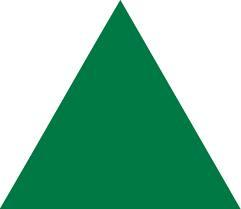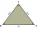# Triangles

Equilateral triangle with side 40 cm has the same perimeter as an isosceles triangle with arm of 45 cm. Calculate the base x of an isosceles triangle.

Result

x =  30 cm

#### Solution:

$x = 3 \cdot 40-2 \cdot 45 = 30 \ \text{cm}$

Try calculation via our triangle calculator.Our examples were largely sent or created by pupils and students themselves. Therefore, we would be pleased if you could send us any errors you found, spelling mistakes, or rephasing the example. Thank you!

Leave us a comment of this math problem and its solution (i.e. if it is still somewhat unclear...):Be the first to comment!Tips to related online calculators
Do you have a linear equation or system of equations and looking for its solution? Or do you have quadratic equation?

## Next similar math problems:

1. Sides of triangleTriangle circumference with two identical sides is 117cm. The third side measures 44cm. How many cms do you measure one of the same sides?
2. Find xSolve: if 2(x-1)=14, then x= (solve an equation with one unknown)
3. NormThree workers planted 3555 seedlings of tomatoes in one dey. First worked at the standard norm, the second planted 120 seedlings more and the third 135 seedlings more than the first worker. How many seedlings were standard norm?
4. Simple equation 8Solve the following equation: 36=-(1+7x)-6(-7-x)
5. Unknown number 11That number increased by three equals three times itself?
6. Forest nurseryIn the forest nursery after winter, they found that 1/10 stems died out of them. For them, they land 193 new spruces. How many spruces are in the forest nursery?
7. ExpressionSolve for a specified variable: P=a+4b+3c, for a
8. GivenGiven 2x =0.125 find the value of x
9. HotelThe hotel has a p floors each floor has i rooms from which the third are single and the others are double. Represents the number of beds in hotel.
10. Simple equation 1035= 7*3*x what is x?
11. Equation 29Solve next equation: 2 ( 2x + 3 ) = 8 ( 1 - x) -5 ( x -2 )
12. Simple equationSolve for x: 3(x + 2) = x - 18
13. Dropped sheetsThree consecutive sheets dropped from the book. The sum of the numbers on the pages of the dropped sheets is 273. What number has the last page of the dropped sheets?
14. Simple equationSolve the following simple equation: 2. (4x + 3) = 2-5. (1-x)
15. SimplifySimplify the following problem and express as a decimal: 5.68-[5-(2.69+5.65-3.89) /0.5]
16. Negative in equation2x + 3 + 7x = – 24, what is the value of x?
17. Simple equation 9Solve the following equation: -8y+5=-9y+9